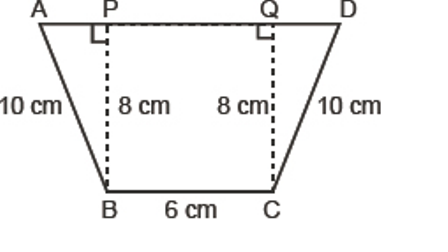Maths / Areas Of Parallelograms And Triangles / Area of Triangle

QUESTION

Find the area of the following quadrilateral.EXPLANATION
Explain TypeExplanation Content
Text

Area of Trapezium ABCD =

We can also calculate by finding area of triangle APB, triangle BCQ, rectangle PQCB.

traingle APB =

triangle BCQ =

rectangle PQCB =

Total Area =

TestimonialsPARENT FEEDBACK c/o Om Umang C/o ABHYAS Academy
10th
Abhyas is a complete education Institute. Here extreme care is taken by teacher with the help of regular exam. Extra classes also conducted by the institute, if the student is weak.